# Minimal Rank Completions

I’m delighted to share that my first applied mathematics paper, Minimal Rank Completions for Overlapping Blocks, coauthored with Nithin Govindarajan and Shivkumar Chandrasekaran, has been accepted for publication in Linear Algebra and its Applications. (We also have a preprint on arXiv.) In this post, I wanted to share what our paper is about and share an alternate derivation which ended up being cut from the final paper.

Our paper is concerned with the following question: given a collection of matrices which overlap in a particular way, can we choose the region of simultaneous overlap so as to minimize the rank of each of the individual matrices? This is a multi-objective optimization problem, so a priori it is not clear it has a simultaneous solution. So it was of great surprise to us when we discovered that it does. In fact, we were able to find a tractable algorithm to find all solutions to this multi-objective problem.

Our enormous thanks go to the anonymous reviewer, who went above and beyond by discovering and sketching to us a dramatically shorter and more revealing proof1In particular, our original proof only produced some solutions to this problem, where the reviewer’s improved proof strategy allowed us to characterize all solutions. of our main theorem. We are truly indebted to this extraordinary reviewer for the significantly streamlined and more understandable presentation in the current iteration of our paper.

For the remainder of this post, I want to discuss minimal rank completions in a little more generality and present a solution to a special case of a minimal rank completion problem which can be tractably solved. I will present a solution to this problem we discovered which I have not seen elsewhere in the literature. I hope that this perspective will be complementary to existing solutions to this problem published in the literature including the summary of this result in our paper.

## Minimal Rank Completion Problems

Minimal rank completions have achieved a lot of buzz in statistics and data science. Given a matrix with unknown entries, it is often very natural to impute the missing entries as those minimizing the rank of the matrix. This is justified by a kind of Occam’s razor argument: big data matrices are very often (approximately) low-rank, so when data is missing, we may assume it takes whatever values are necessary to minimize the rank.

Alternately, if the missing entries belong to a special pattern, it may be possible to find the rank-minimizing choice of the unknown entries using linear algebraic tools like matrix factorizations. These approaches have significantly more limited domains of applicability than optimization-based approaches, but have advantages in that they

• can be more tractable as they involve matrix factorizations rather than solving optimization problems;
• work over arbitrary fields of numbers, such as finite fields appearing in computer science;
• can find all ways of choosing the entries to minimize the rank, in particular certifying or dis-certifying the uniqueness of the rank-minimizing choice; and
• are guaranteed to find the rank-minimizer, without any statistical assumptions.

These algebraic methods have their roots in systems theory, integral operators, and rank-structured matrices. The last of these was the application which motivated our interest in the subject.

Our paper concerns an overlapping variant of this problem, where we simultaneously minimize the ranks of several matrices by choosing the entries in the overlap carefully. This problem emerged to us naturally in the construction of certain matrix representations, and we hope it might prove useful in tackling other problems. As it turns out, the overlapping problem can be solved very much in the spirit of a much simpler problem, the block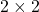minimal rank completion problem, which we will spend most of the remainder of this post discussing.

## A Solution to the Block 2×2 Case

The blockminimal rank completion problem is as follows: given a partially filled block matrix

(1)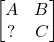how can the “?” be filled in to minimize the rank of this matrix?

A generalized version of this problem was originally solved by Kaashoek and Woerdeman. An alternate derivation using matrix factorizations is given by Eidelman, Gohberg, and Haimovici, though they only find some of the solutions to this problem. We seek to characterize all ways of choosing the “?” so that the rank is minimize the rank.

Here, I present the solution to this problem which my coauthors and I originally discovered, which is different than the one we summarize in the final version of our paper.2This construction does appear in Section 4 of an initial preprint of ours on rank-structured matrices. This solution is in the spirit of the one by Eidelman, Gohberg, and Haimovici but does produce all solutions.

Let’s start by recalling some facts about linear algebra. Given an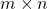real3The analysis in this discussion holds over any field, but we shall use the real numbers for concreteness. matrix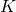, one can define its column space, which I will denote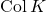, to be the vector space consist of all linear combinations of the columns of. From this column space, or indeed any vector subspace of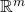, one can extract a basis for this subspace, meaning every vector in the subspace can be uniquely decomposed as a linear combination of elements from the basis. In this discussion, we shall always arrange the basis vectors as columns of a basis matrix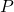. If we instead consider the row space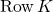of, then we arrange the elements of a basis as rows of a matrix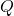.

Before we solve the problem, let’s reason about the lowest possible rank we could possibly expect for the completed matrix. Since the rank of a matrix can be no smaller than the rank of any of its submatrices, clearly we must have that, for any assignment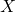of the “?”,

(2)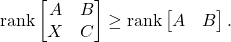However, in general, the rank of the completed matrix must be higher than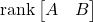, as is exemplified when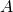and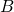are both zero but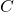is not. In addition to rank of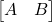, we must also account for rows of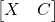which cannot be written as linear combinations of the rows above, no matter howis chosen. With a little noodling, one can convince oneself that there are always at least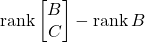such rows, leading to the bound

(3)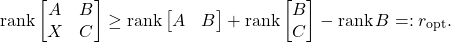We shall show that, by judicious choice of, Eq. (3) can always be achieved with equality, making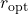the minimal rank for this completion problem.

Let us now find such a rank-minimizing. The construction begins by considering the column spaces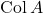and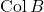of the matricesand. The intersection of these column spaces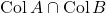is again a vector subspace, and we choose a basis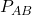for it. The subspacemight have a smaller size than. Therefore, to find a basis for, we can extend the basisby adding new columns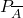to it so that the enlarged matrix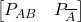forms a basis for. Similarly, we can find new columns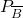to add toso that the matrix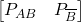forms a basis for.

Now comes something subtle. Since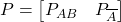forms a basis for, every column incan be written as a linear combination of columns in. In matrix language, this means there exists a matrix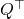such that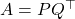—in fact, this exactforms a basis for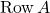. Sinceis divided into two collections of columns, it is only natural to similarly (and conformally) divideinto two pieces as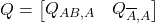. Thus, doing the same withas we did with, we obtain two matrix factorizations

(4)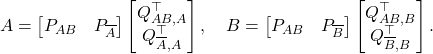Now we do the same song and dance with the row spaces ofand. Let’s go through the construction somewhat quickly. We start with a basis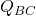for the intersection of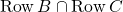. We then extend these to bases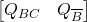and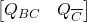for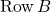and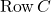. From here, we derive the existence of matrix factorizations analogous to Eq. (4):

(5)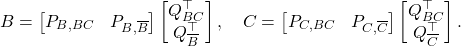For the next part, we shall take advantage of a powerful fact: if I have a basesandfor the row and column spaces of a matrix, there exists a nonsingular matrix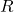for which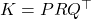. Applying this fact to the bases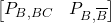and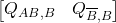for‘s column and row spaces, we get the existence of a matrix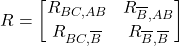such that

(6)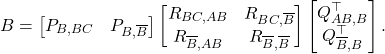We now have all ingredients to describe the solution. Rather than trying to “derive” the solution in a rigorous way, let us try and discover the solution in a non-rigorous way and justify our work later. We’re hoping to find assignmentsof the “?” such that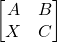achieves the minimum possible rankdefined in Eq. (3). To do so, let’s try and find a rank factorization of the completed matrix and then infer what valueswill take. First off, let’s build a rank factorization for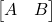using the building blocks we’ve already built

(7)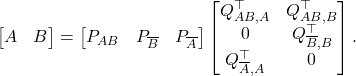Now we want to extend this to a rank factorization for the entire completed matrix. Let’s build up to this in stages, denoting by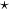entries whose value we have yet to determine. For us to have a hope of representing the matrix, we’ll need to somehow add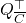into our rank factorization. Doing exactly this, we get the partially specified rank factorization

(8)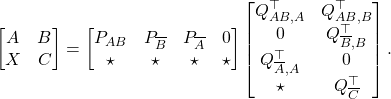Now, to fill in the second block row of the left factor, we recall that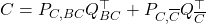. From Eqs. (5) and (6), we deduce that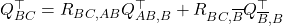. Thus, we can fill in more entries:

(9)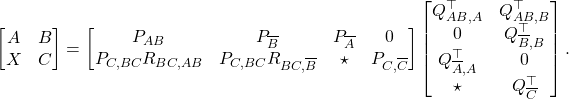With these entries filled in, the remaining‘s can be chosen arbitrarily. Assigning names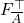and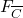to these free variables, we conclude that

(10)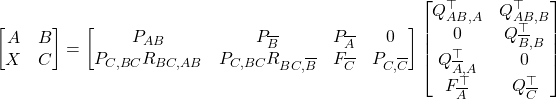and

(11)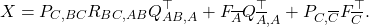From all the analysis previous, we know that all‘s of the form Eq. (11) solve the minimal rank completion problem, making the completed matrix achieve the minimal rankdefined in Eq. (3). With a little more elbow grease, one can also confirm that all such minimal completionsare of the form Eq. (11), proving that Eq. (11) indeed characterizes all solutions to the minimal rank completion problem. This completes the characterization and construction of the complete set of minimizers to the blockminimal rank completion problem.

## The Overlapping Block Minimal Rank Completion Problem

If you found this post interesting, be sure to check out our paper (or here on arXiv) for an explanation of a different way of thinking about the solution of the blockminimal rank completion problem and a solution to a more general “overlapping” variant. The treatment should be reasonably self-contained, and we hope the solution to this problem could prove a useful tool in tackling open problems in systems theory and the study of rank-structured matrices.